## Parameter

A parameterused in Elliptic Integrals defined to be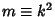, where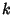is the Modulus. An Elliptic Integral is written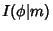when the parameter is used. The complementary parameter is defined by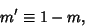(1)

whereis the parameter. Let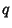be the Nome,the Modulus, andthe Parameter. Then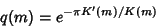(2)

where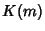is the complete Elliptic Integral of the First Kind. Then the inverse of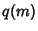is given by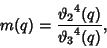(3)

where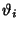is a Theta Function.

See also Amplitude, Characteristic (Elliptic Integral), Elliptic Integral, Elliptic Integral of the First Kind, Modular Angle, Modulus (Elliptic Integral), Nome, Parameter, Theta Function

References

Abramowitz, M. and Stegun, C. A. (Eds.). Handbook of Mathematical Functions with Formulas, Graphs, and Mathematical Tables, 9th printing. New York: Dover, p. 590, 1972.